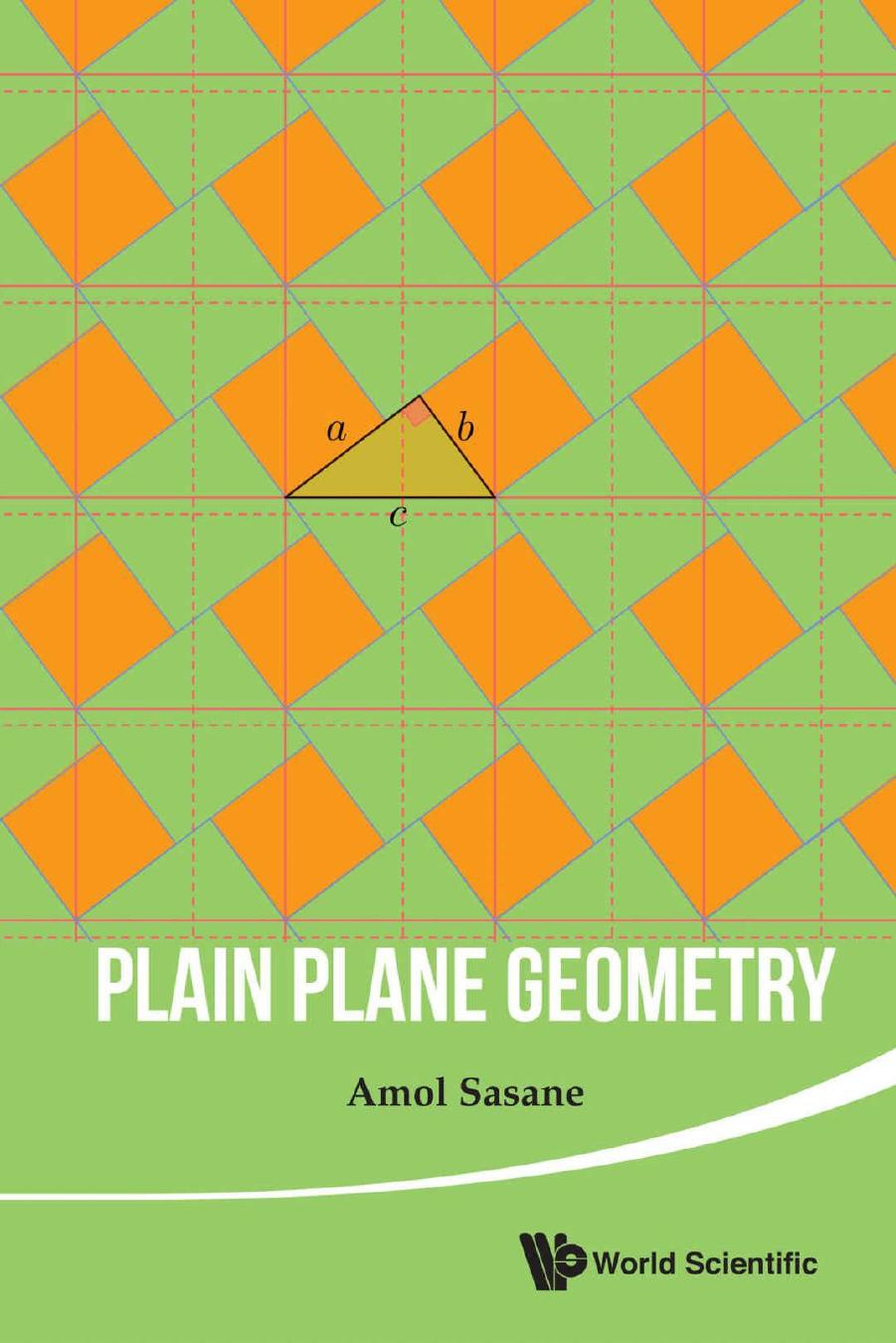# Plain Plane Geometry by Amol SasaneAuthor:Amol Sasane [Sasane, Amol]
Language: eng
Format: azw3
ISBN: 9789814740463
Publisher: WSPC
Published: 2015-12-07T05:00:00+00:00

By constructing the right angled triangle with nonhypotenuse side lengths AB and 2AB, we obtain the length as the length of the hypotenuse. Hence we can also construct (by adding the length AB to the length , and bisecting the resulting line segment) the length x.

Now the construction of the pentagon ABCDE is straightforward:

(1)

With A as center and radius x, draw a circle C(A, x). Similarly, draw the circle C(B, x). Let D be one of the intersection points of C(A, x) and C(B, x).

(2)

On the same side of AB as the point D, let C be the intersection point of the circle C(A, x) and C(B, AB), and let E be the intersection point of the circle C(B, x) and C(A, AB).